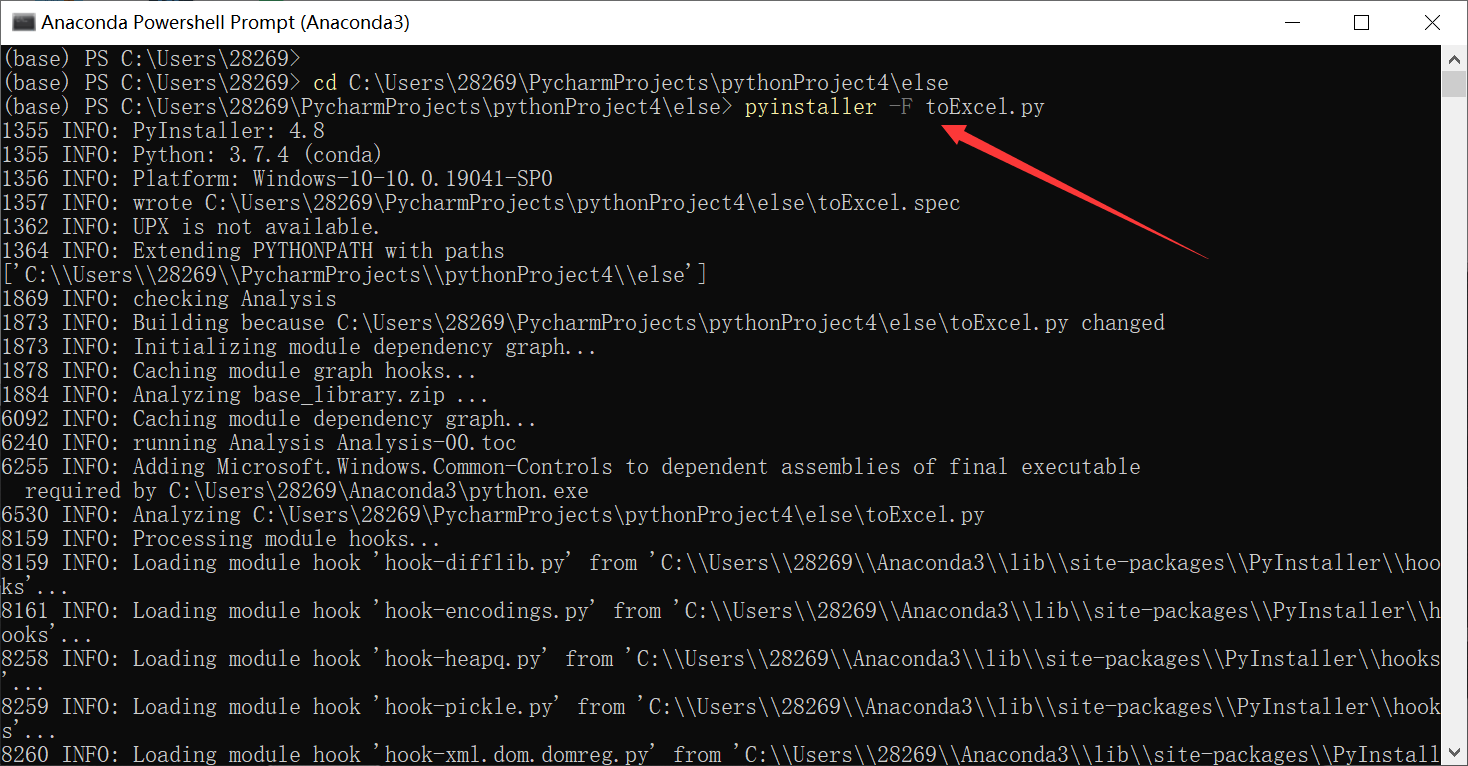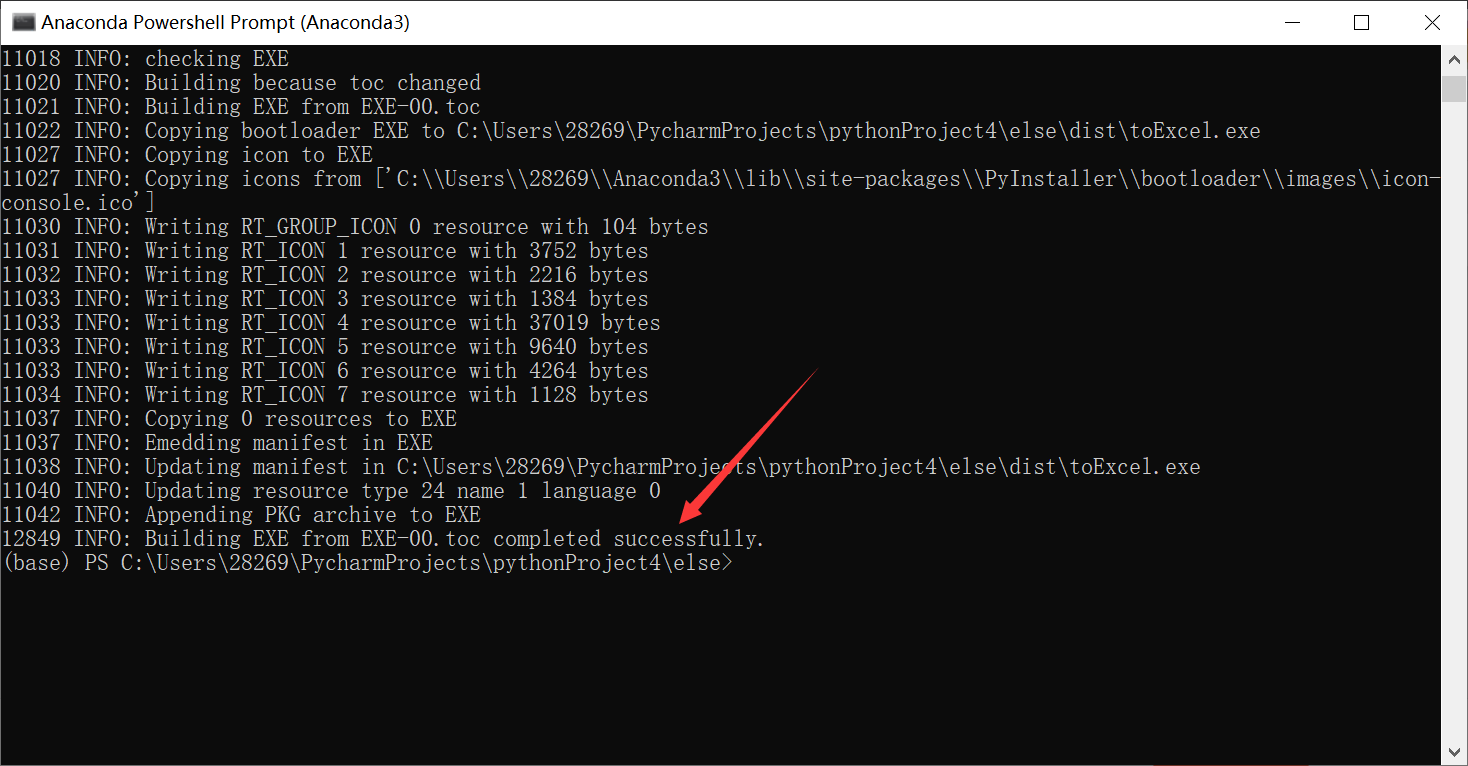# Python针对Excel数据的处理（部分）

1.xlrd

2.xlwt

3.xlutils

1.在Excel中通过列名称对整列数据进行索引

2.对嵌套列表中的元素进行索引

3.定义一个函数，寻找列表中所有.xls和.xlsx文件

4.释放内存，提升cpu性能，删除已创建的对象

5.运行程序时，使黑框不自动关闭

# 1.xlrd

1.导入函数xlrd

前提是已安装xlrd模块。

♦到python官网​​​​​​http://pypi.python.org/pypi/xlrd下载模块安装，前提是已经安装了python 环         境。

♦或者在cmd窗口  pip install  xlrd

``import xlrd``

2.xlrd读取Excel文件：

``data=xlrd.open_workbook("文件名")``

3.data处理

（1）.获取Excel中的一个sheet表：

``````table = data.sheets()                 #通过索引顺序获取

table = data.sheet_by_index(sheet_indx)) #通过索引顺序获取

table = data.sheet_by_name(sheet_name)   #通过名称获取
``````

（2）.对sheet中数据的处理需要进行行列索引：

``````nrows = table.nrows #获取该sheet中的有效行数

table.row(rowx) #返回由该行中所有的单元格对象组成的列表

table.row_slice(rowx) #返回由该列中所有的单元格对象组成的列表

table.row_types(rowx, start_colx=0, end_colx=None) #返回由该行中所有单元格的数据类型组成的列表

table.row_values(rowx, start_colx=0, end_colx=None) #返回由该行中所有单元格的数据组成的列表

table.row_len(rowx) #返回该列的有效单元格长度``````

# 2.xlwt

1.导入函数xlwt：

``import xlwt``

2.通过xlwt对Excel中的单元格进行颜色标记：

``````import xlwt
workbook = xlwt.Workbook()
pattern = xlwt.Pattern() # Create the Pattern
pattern.pattern = xlwt.Pattern.SOLID_PATTERN # May be: NO_PATTERN, SOLID_PATTERN, or 0x00 through 0x12
pattern.pattern_fore_colour = 5 # May be: 8 through 63. 0 = Black, 1 = White, 2 = Red, 3 = Green, 4 = Blue, 5 = Yellow, 6 = Magenta, 7 = Cyan, 16 = Maroon, 17 = Dark Green, 18 = Dark Blue, 19 = Dark Yellow , almost brown), 20 = Dark Magenta, 21 = Teal, 22 = Light Gray, 23 = Dark Gray, the list goes on...
style = xlwt.XFStyle() # Create the Pattern
style.pattern = pattern # Add Pattern to Style
worksheet.write(0, 0, 'Cell Contents', style)
workbook.save('Excel_Workbook.xls')
``````

# 3.xlutils

1. 读取表格信息的功能
2. 在表格中写入数据的功能

1.导入函数：

``from xlutils.copy import copy``

2.函数使用：

``new_workbook=copy(old_workbook)``

# 二、程序中的各种方法实现

## 1.在Excel中通过列名称对整列数据进行索引

``````def getColumnIndex(table, columnName):
columnIndex = None
for i in range(table.ncols):
if (table.cell_value(0, i) == columnName):
columnIndex = i
break
return columnIndex``````

## 2.对嵌套列表中的元素进行索引

``````lst=[["a", "b", "c"], ["d", "e", "f"], ["g","h"]]
check="a"
print([[index1,index2] for index1,value1 in enumerate(lst) for index2,value2 in enumerate(value1) if value2==check])``````

# 3.定义一个函数，寻找列表中所有.xls和.xlsx文件

``````def get_all_excel(dir):
file_list = []
for root_dir, sub_dir, files in os.walk(r'' + dir):
# 对文件列表中的每一个文件进行处理，如果文件名字是以‘xlxs’结尾就
# 认定为是一个excel文件，当然这里还可以用其他手段判断，比如你的excel
# 文件名中均包含‘res’，那么if条件可以改写为
for file in files:
# if file.endswith('.xlsx') and 'res' in file:
if file.endswith('.xls') or file.endswith('.xlsx'):
# 此处因为要获取文件路径，比如要把D:/myExcel 和res.xlsx拼接为
# D:/myExcel/res.xlsx，因此中间需要添加/。python提供了专门的
# 方法
file_name = os.path.join(root_dir, file)
# 把拼接好的文件目录信息添加到列表中
file_list.append(file_name)
return file_list``````

# 4.释放内存，提升cpu性能，删除已创建的对象

``````sheet.release_resources()
del sheet``````

# 5.将源代码打包成.exe文件

pyinstaller安装的两种方法：

（1）.打开命令提示符（搜索栏输入cmd），使用pip命令安装pyinstaller

（2）.使用Anaconda内置的控制面板安装pyinstaller# 6.运行程序时，使黑框不自动关闭

``````import os.path
import os
os.system("pause")``````